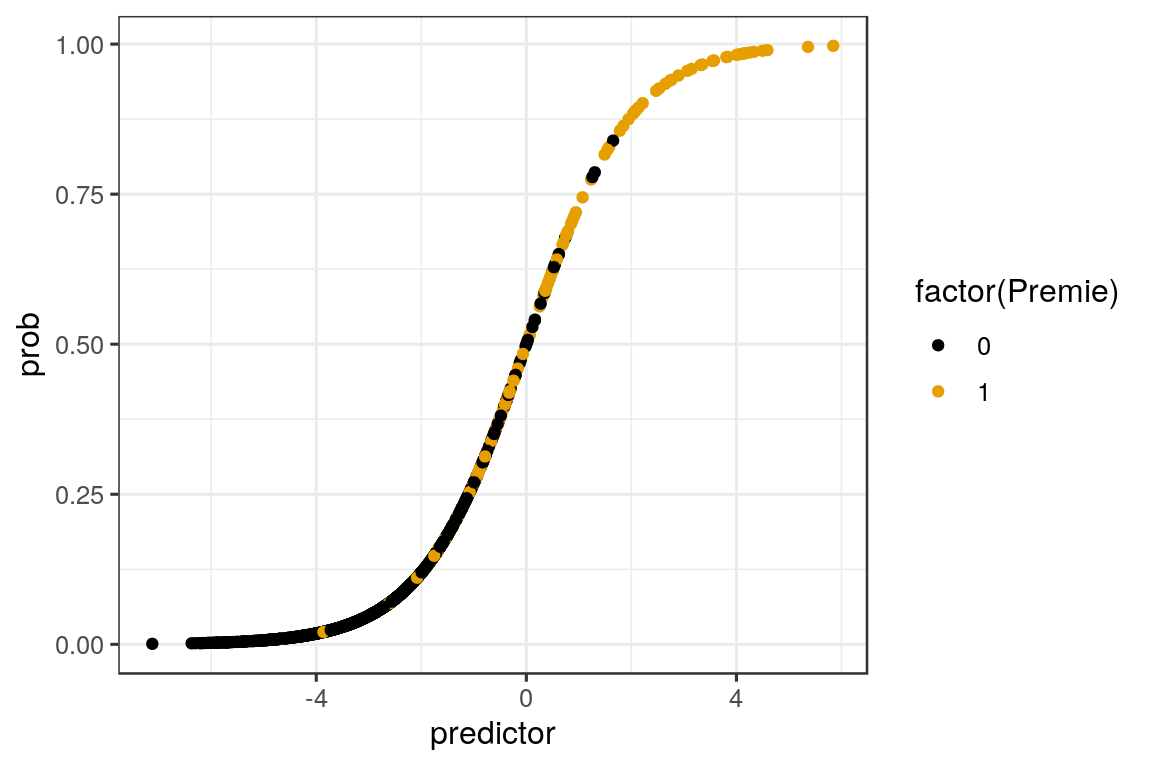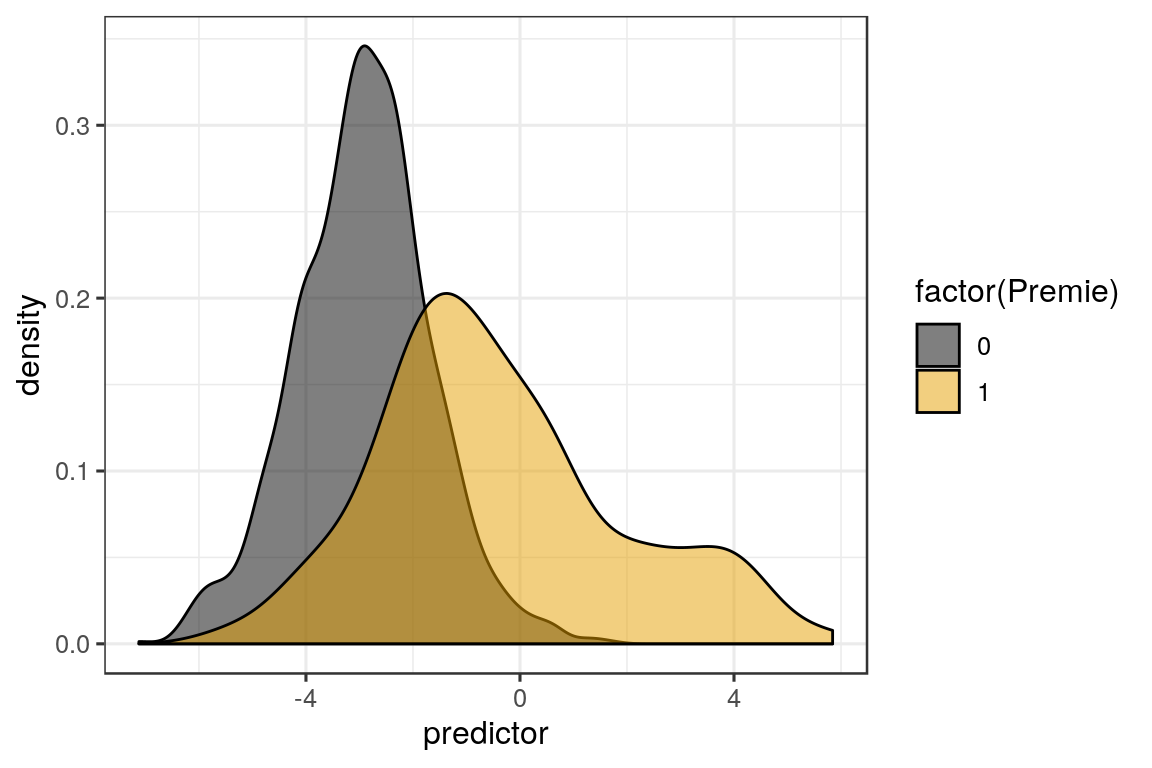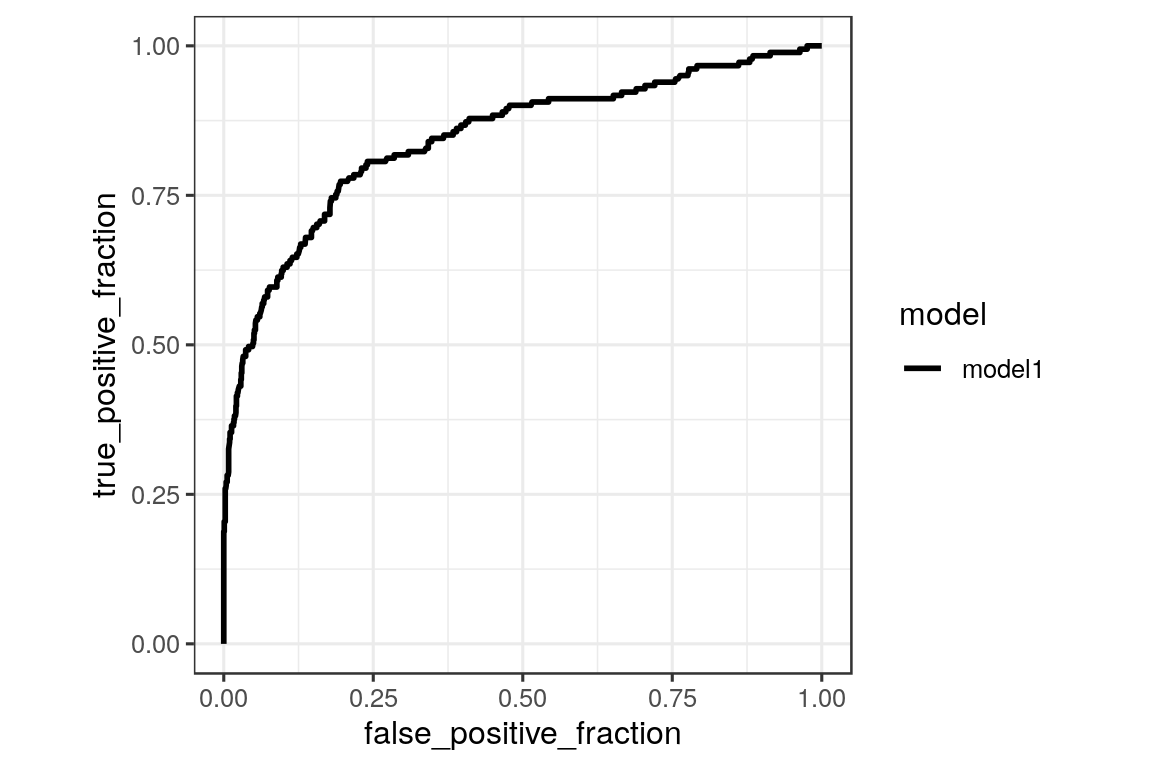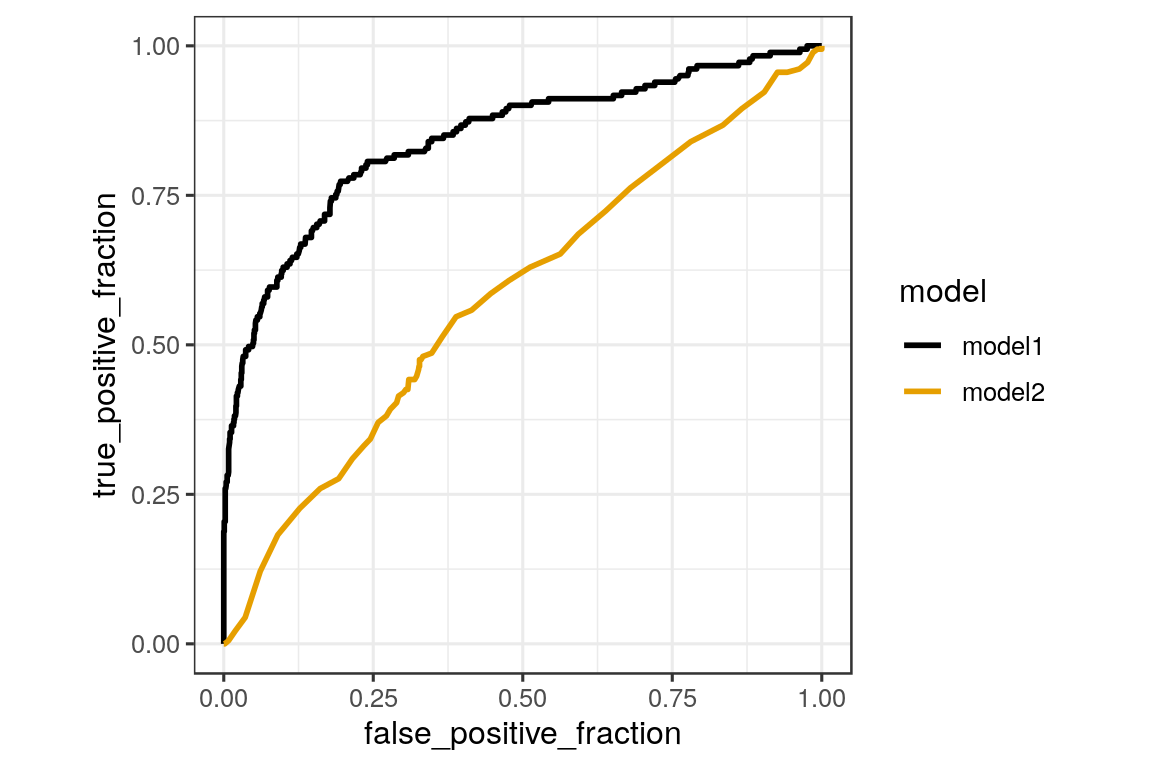## Homework 6

Enter your name and EID here

This homework is due on Mar. 9, 2020 at 12:00pm. Please submit as a PDF file on Canvas.

For this homework, you will work with a dataset collected by John Holcomb from the North Carolina State Center for Health and Environmental Statistics. This data set contains 1409 birth records from North Carolina in 2001.

NCbirths <- read_csv("http://wilkelab.org/classes/SDS348/data_sets/NCbirths.csv")
## Parsed with column specification:
## cols(
##   Plural = col_double(),
##   Sex = col_double(),
##   MomAge = col_double(),
##   Weeks = col_double(),
##   Gained = col_double(),
##   Smoke = col_double(),
##   BirthWeightGm = col_double(),
##   Low = col_double(),
##   Premie = col_double(),
##   Marital = col_double()
## )
head(NCbirths)
## # A tibble: 6 x 10
##   Plural   Sex MomAge Weeks Gained Smoke BirthWeightGm   Low Premie Marital
##    <dbl> <dbl>  <dbl> <dbl>  <dbl> <dbl>         <dbl> <dbl>  <dbl>   <dbl>
## 1      1     1     32    40     38     0         3147.     0      0       0
## 2      1     2     32    37     34     0         3289.     0      0       0
## 3      1     1     27    39     12     0         3912.     0      0       0
## 4      1     1     27    39     15     0         3856.     0      0       0
## 5      1     1     25    39     32     0         3430.     0      0       0
## 6      1     1     28    43     32     0         3317.     0      0       0

The column contents are as follows:

• Plural: 1=single birth, 2=twins, 3=triplets.
• Sex: sex of the baby 1=male 2=female.
• MomAge: Mother’s age (in years).
• Weeks: Completed weeks of gestation.
• Gained: Weight gained during pregnancy (in pounds).
• Smoke: Mother is a smoker: 1=yes, 0=no.
• BirthWeightGm: Birth weight in grams.
• Low: Indicator for low birth weight, 1=2500 grams or less, 0=otherwise.
• Premie: Indicator for premature birth, 1=36 weeks or sooner, 0=otherwise.
• Marital: Marital status: 0=married or 1=not married.

Problem 1: (5 pts)

a. (1 pt) Make a logistic regression model that predicts premature births (Premie) from birth weight (BirthWeightGm), plural births (Plural), and weight gained during pregnancy (Gained) in the NCbirths data set. Show the summary (using summary()) of your model below.

glm_out <- glm(Premie ~ Plural + BirthWeightGm + Gained,
data = NCbirths,
family = "binomial")

summary(glm_out)
##
## Call:
## glm(formula = Premie ~ Plural + BirthWeightGm + Gained, family = "binomial",
##     data = NCbirths)
##
## Deviance Residuals:
##     Min       1Q   Median       3Q      Max
## -1.9120  -0.4410  -0.2964  -0.1693   3.3480
##
## Coefficients:
##                 Estimate Std. Error z value Pr(>|z|)
## (Intercept)    4.8586938  0.7962407   6.102 1.05e-09 ***
## Plural         0.6713701  0.3779965   1.776   0.0757 .
## BirthWeightGm -0.0025932  0.0002102 -12.335  < 2e-16 ***
## Gained         0.0130966  0.0073036   1.793   0.0729 .
## ---
## Signif. codes:  0 '***' 0.001 '**' 0.01 '*' 0.05 '.' 0.1 ' ' 1
##
## (Dispersion parameter for binomial family taken to be 1)
##
##     Null deviance: 1080.56  on 1408  degrees of freedom
## Residual deviance:  745.54  on 1405  degrees of freedom
## AIC: 753.54
##
## Number of Fisher Scoring iterations: 6

b. (1 pt) Make a plot to show how the model separates premature births from regular births. Your plot should use the the fitted probabilities and/or the linear predictors, and you should color your geom by the indicator of premature births.

model_data <- data.frame(predictor = glm_out$linear.predictors, prob = glm_out$fitted.values, NCbirths)

ggplot(model_data, aes(x = predictor, y = prob, color = factor(Premie))) +
geom_point() +
scale_color_colorblind()ggplot(model_data, aes(x = predictor, fill = factor(Premie))) +
geom_density(alpha = 0.5) +
scale_fill_colorblind()c. (3 pts) Use the probability cut-off of 0.50 to classify a birth as premature or non-premature. Calculate the true positive rate and the false positive rate and interpret these rates in the context of the NCbirths dataset. Your answer should mention something about premature births and the three predictors in part a.

# make a data-frame with probabilities and premature births
pred_data <- data.frame(probability = glm_out$fitted.values, Premie = NCbirths$Premie)

# cutoff of 0.50
cutoff <- 0.50

# number of premature babies identified as premature (true positives)
premie_true <- pred_data %>%
filter(probability > cutoff & Premie == 1) %>%
tally()

# number of babies carried to term identified as babies carried to term (true negatives)
fullterm_true <- pred_data %>%
filter(probability <= cutoff & Premie == 0) %>%
tally()

# total number of premature babies (known postives)
premie_total <- pred_data %>%
filter(Premie == 1) %>%
tally()

# total number of babies carried to term (known negatives)
term_total <- pred_data %>%
filter(Premie == 0) %>%
tally()

# true positive rate
tp <- premie_true$n / (premie_total$n)
tp
##  0.3646409
# true negative rate
tn <- fullterm_true$n / (term_total$n)
tn
##  0.9845277
# false positive rate (1 - true negative rate)
(1 - tn)
##  0.01547231

The true positive rate is 36.5%. The false positive rate is 1.5%. With a probability cut-off of 0.50, our regression model based on birth weight, plural births, and weight gained during pregnancy correctly classifies 36.5% of premature babies. The model incorrectly classifies 1.5% of babies caried to term as premature babies.

Problem 1: (5 pts)
a. (1 pt) Plot an ROC curve for the model that you created in problem 1a. Does the model perform better than a model in which you randomly classify a birth as premature or non-premature? Explain your answer in 1-2 sentences.

HINT: Random classification would lie along y = x.

df1 <- data.frame(
predictor = predict(glm_out, NCbirths),
known_truth = NCbirths$Premie, model = "model1" ) # plot # the aesthetic names are not the most intuitive # d holds the known truth # m holds the predictor values ggplot(df1, aes(d = known_truth, m = predictor, color = model)) + geom_roc(n.cut = 0) + coord_fixed() + scale_color_colorblind()The model I created in problem 1a performs better than a random classifier since the ROC curve is above the y = x line. The true positive rate is always greater than the false positive rate. No matter what probability cut-off we use, the model correctly classifies premature babies more often than it incorrectly classifies non-premature babies as premature. b. (4 pts) Use the mothers’ marital status (Marital) and the mothers’ age (MomAge) as a new set of predictor variables for premature births, and create a logistic regression model. Plot an ROC curve for your newly-created model and, on the same plot, add an ROC curve from your model in problem 1a. What can you conclude from your plot? Which model performs better and why? Support your conclusions with AUC values for each model. glm_out2 <- glm(Premie ~ Marital + MomAge, data = NCbirths, family = "binomial") df2 <- data.frame( predictor = predict(glm_out2, NCbirths), known_truth = NCbirths$Premie,
model = "model2"
)

df_combined <- bind_rows(df1, df2)
## Warning in bind_rows_(x, .id): Unequal factor levels: coercing to character
## Warning in bind_rows_(x, .id): binding character and factor vector,
## coercing into character vector

## Warning in bind_rows_(x, .id): binding character and factor vector,
## coercing into character vector
# plot

# the aesthetic names are not the most intuitive
# d holds the known truth
# m holds the predictor values
p <- ggplot(df_combined, aes(d = known_truth, m = predictor, color = model)) +
geom_roc(n.cuts = 0) +
coord_fixed() +
scale_color_colorblind()
p# calculate AUC
calc_auc(p)
##   PANEL group       AUC
## 1     1     1 0.8445278
## 2     1     2 0.5812623
model <- unique(df_combined\$model)
model_info <- data.frame(
model,
group = order(model)
)

left_join(model_info, calc_auc(p)) %>%
select(-group, -PANEL) %>%
arrange(desc(AUC))
## Joining, by = "group"
##    model       AUC
## 1 model1 0.8445278
## 2 model2 0.5812623

The newly-created model performs worse than the model created in problem 1a because the AUCs are 0.58 and 0.84, respectively. I conclude that birth weight, plural births, and weight gained during pregnancy are, collectively, better predictors of premature births than the mothers’ marital status and the mothers’ age.